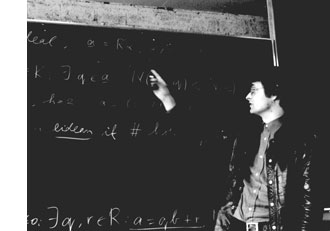Here’s a 5 move game from $\mathbb{C}$, the complex numbers game, annotated by Hendrik Lenstra in Nim multiplication.

$\begin{matrix} & \text{White} & \text{Black} \\ 1. & 3-2i & { 3_{\mathbb{R}} } \\ 2. & 3_{\mathbb{R}} & (22/7)_{\mathbb{Q}} \\ 3. & (-44_{\mathbb{Z}},-14_{\mathbb{Z}})? & { -44_{\mathbb{Z}} } \\ 4. & -44_{\mathbb{Z}} & ( 0_{\mathbb{N}},44_{\mathbb{N}} )! \\ 5. & \text{Resigns} & \\ \end{matrix}$

He writes : “The following 5 comments will make the rules clear.

1 : White selected a complex numbers. Black knows that $\mathbb{C} = \mathbb{R} \times \mathbb{R}$ by $a+bi = (a,b)$, and remembers Kuratowski’s definition of an ordered pair: $~(x,y) = { { x }, { x,y } }$. Thus black must choose an element of ${ { 3_{\mathbb{R}} }, { 3_{\mathbb{R}},-2_{\mathbb{R}} } }$. The index $\mathbb{R}$ here, and later $\mathbb{Q},\mathbb{Z}$ and $\mathbb{N}$, serve to distinguish between real numbers, rational numbers, integers and natural numbers usually denoted by the same symbol. Black’s move leaves White a minimum of choice, but it is not the best one.

2 : White has no choice. The Dedekind definition of $\mathbb{R}$ which the players agreed upon identifies a real number with the set of all strictly larger rational numbers; so Black’s move is legal.

3 : A rational number is an equivalence class of pairs of integers $~(a,b)$ with $b \not= 0$; here $~(a,b)$ represents the rational number $a/b$. The question mark denotes that White’s move is a bad one.

4 : The pair $~(a,b)$ of natural numbers represents the integer $a-b$. Black’s move is the only winning one.

5 : White resigns, since he can choose between ${ 0_{\mathbb{N}} }$ and ${ 0_{\mathbb{N}},44_{\mathbb{N}} }$. In both cases Black will reply by $0_{\mathbb{N}}$, which is the empty set” (and so wins because White has no move left).

These rules make it clear what we mean by the natural numbers $\mathbb{N}$ game, the $\mathbb{Z}$-game and the $\mathbb{Q}$ and $\mathbb{R}$ games. A sum of games is defined as usual (players are allowed to move in exactly one of the component games).

Here’s a 5 term exercise from Lenstra’s paper : Determine the unique winning move in the game $\mathbb{N} + \mathbb{Z} + \mathbb{Q} + \mathbb{R} + \mathbb{C}$

It will take you less than 5 minutes to solve this riddle. Some of the other ‘exercises’ in Lenstra’s paper may take you a lot longer, if not forever…

Exactly 5 years ago I wrote : “As it is probably better to run years behind than to stand eternally still, I’ll try out how much of a blogger I am in 2004.”

5 months ago this became : “from january 1st 2009, I’ll be moving out of here. I will leave the neverendingbooks-site intact for some time to come, so there is no need for you to start archiving it en masse, yet.”

5 minutes before the deadline, this will be my last post….

of 2008

less entropy in 2009!

Published in web